# Most Frequently Asked Accounting Questions Students Usually Get Wrong

## Learning Accounting isn't Easy!

Most accounting classes start with teaching basic accounting concepts which should give you the ability to answer the questions you'll see on your homework, quizzes, and tests. The idea is to build a foundation which allows you to successfully navigate and answer the more complex problems you'll encounter later on in your accounting class. That's handy, right? Unfortunately, some of the questions on your accounting quizzes and tests can derail your best efforts...you think you know the information and then the quiz/test kicks your butt!

When students receive accounting help from me, we focus on working accounting practice problems. These are similar to the questions and answers you'll see on your quizzes and exams... the tricky ones that really make you think... because this is what trips most accounting students up. It's the problem with most accounting classes. When homework is usually in a different format than the test or quiz questions, it's hard to make the connection between what you learned from your homework and what you need to do to pass your test. And this is one of the reasons why accounting students get good grades on homework and bad grades on the test/quiz.

The truth is, if you were to ask an accountant how to solve your test or quiz questions, they may struggle too. Arriving at accounting answers is a process that once learned, can make accounting easier. It's like learning a secret that unlocks a new way of thinking; you'll see things completely differently. I provide accounting help online and in person, but I mainly tutor students over the internet using online conferencing tools like zoom. Over the years I've seen where students struggle and where they succeed. More importantly, I see why they struggle and why they succeed. It has to do with how they study.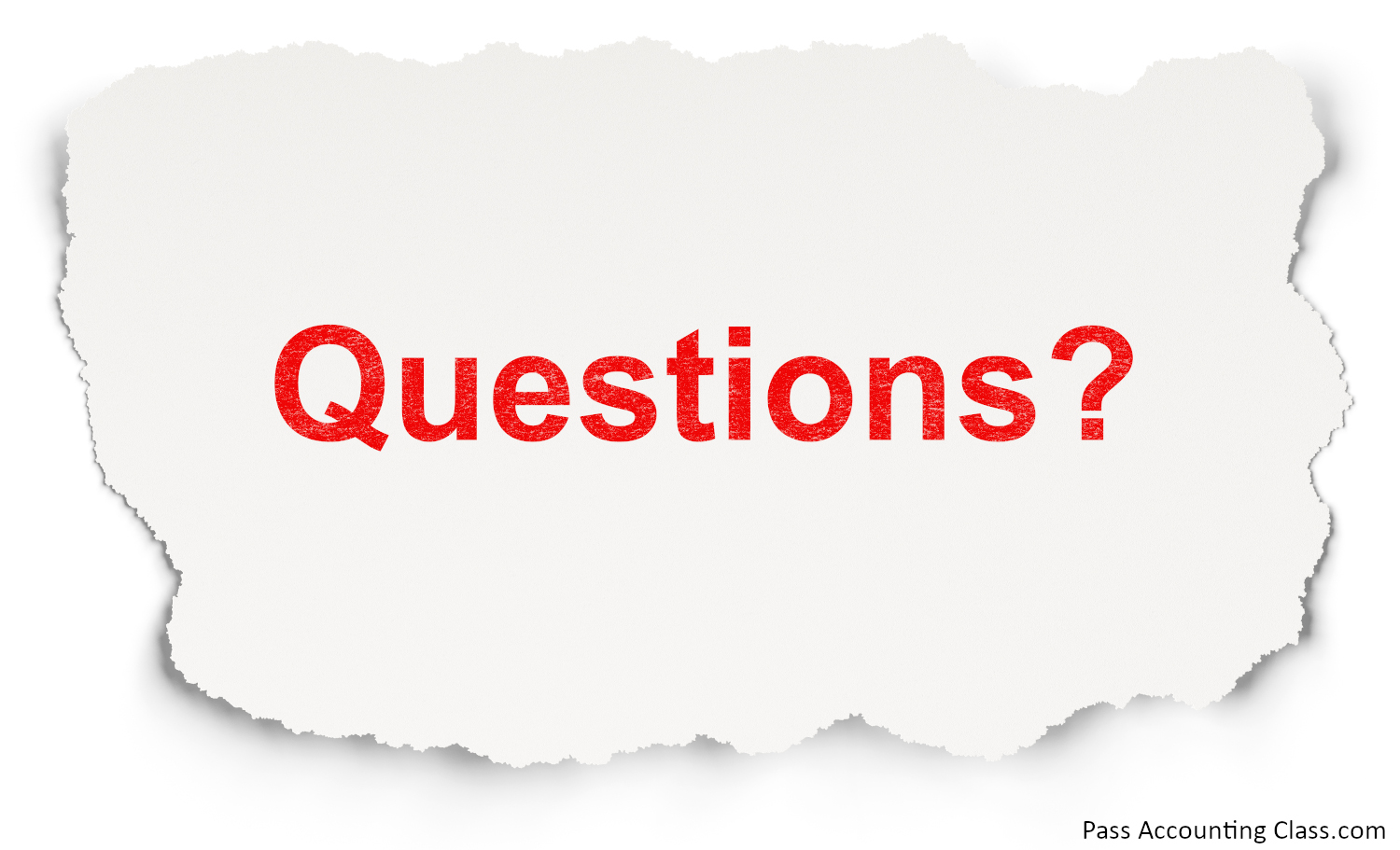## Practice Problems

Successful students quickly realize the difference between basic accounting questions and more complex accounting problems. They focus on what they don't know and figure out how to learn it. One of the methods that I teach is to look at the accounting answers and understand how to arrive at that answer. This is often referred to as "reverse engineering." Basically, you figure out how to get the answer from the information given in the problem. This helps you establish a system that can be applied to similar questions. Over time, you learn the system, which in turn allows you to answer the questions correctly, and quickly. Unfortunately, books and professors don't always show you these "systems."

As I mentioned earlier, the problems you'll see on an accounting quiz or an accounting test are usually in a different format than your homework questions. This is where I see a disconnect and it's what causes students to do poorly on their test/quiz. My goal and focus in offering accounting help online is to show my students a system that helps them answer even the most challenging accounting questions efficiently. Imagine how it would be to go from feeling like there's never enough time to finish your tests to answering all the questions with time to spare! You'll be less stressed about your tests and quizzes and get better grades!

## Questions and Answers for Accounting Exams

The five questions below are examples of the most frequently asked accounting questions students usually get wrong. These are very similar to the questions and answers for accounting exams you'll have in your classes. The questions are somewhat tricky, but will be a breeze once you learn the system I teach in Pass Accounting Class.

#### Question 1

Smith Co. reported \$750,000 in Sales, \$325,000 in Cost of Goods Sold, and Net Income of \$150,000. What were Smith Co.’s Operating Expenses?
A) \$425,000
B) \$275,000
C) \$475,000
D) \$325,000

For this question, you need to understand the relationship of numbers on an Income Statement. More specifically, Net Income = Sales - Cost of Goods Sold - Operating Expenses. Here's the first step to solving this problem, writing it out: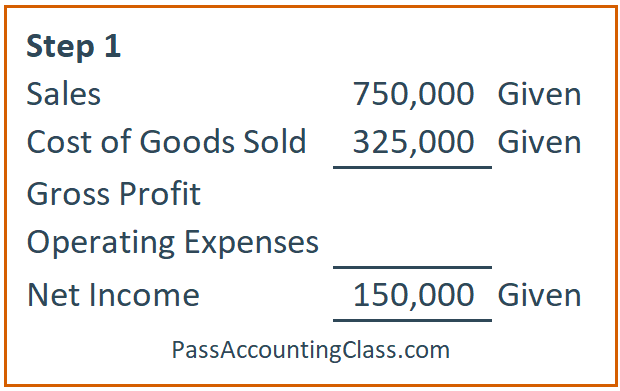The second step is to solve for the Gross Profit: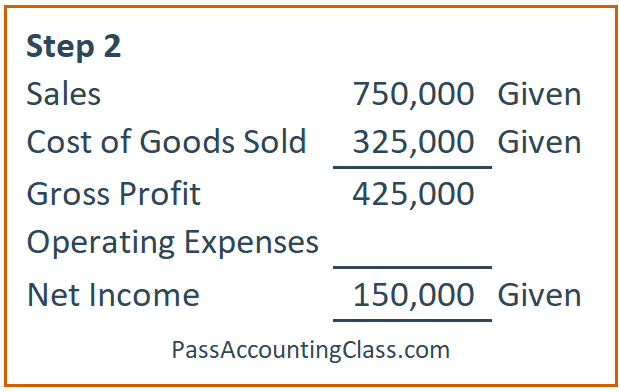The third and final step is to solve for the Operating Expenses by subtracting the Net Income from the Gross Profit: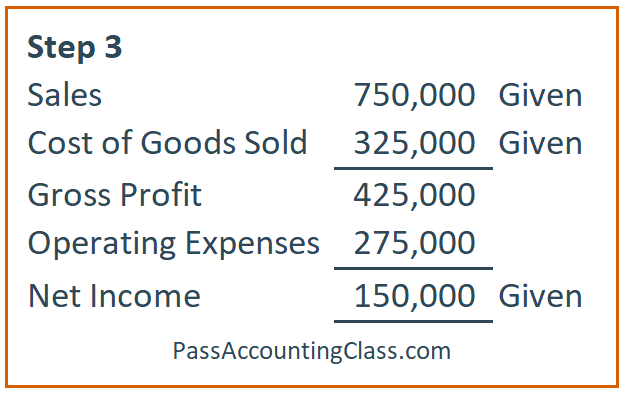Based on using these three steps we know the Operating Expenses are \$275,000. So the answer to the first question is B.

#### Question 2

In a period with increasing prices, which inventory cost flow assumption results in the highest ending inventory value?A) Average Cost
B) FIFO
C) LIFO
D) None of the above

This is one of those accounting problems that is very common. It's a "theory" question and will either be easy for you or give you a headache! I'm not good with theory questions, so I prefer to plug numbers in to solve a question like this.
So, the first step is to draw out increasing prices and assign FIFO (First in, First out) and LIFO (Last in, Last out). Here's what step one looks like: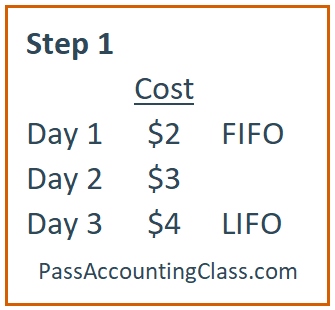For step two we need to find our Ending Inventory (EI) number for FIFO and LIFO. So, we need to identify the Cost of Goods Sold (COGS) for FIFO and LIFO and then subtract the total cost fro the COGS to arrive at our Ending Inventory. Here's what that looks like: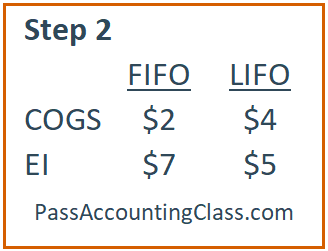Ending Inventory under FIFO is calculated by adding Day 2 and Day 3 (\$3 + \$4) and Ending Inventory under LIFO is calculated by adding Day 1 and Day 2 (\$2 + \$3). Based on using these two steps we know the FIFO produces the highest ending inventory value in a period with increasing prices. So the answer to the second question is B.

#### Question 3

At the beginning of the year, Smith Co. reported assets of \$500,000 and liabilities of \$375,000. During the year, they did not pay dividends, they had \$83,000 in sales, and \$65,000 in expenses. Smith Co. had an increase in total assets of \$25,000 and their liabilities decreased by \$3,000. What was the amount of common stock issued during the year?
A) \$3,000
B) \$7,000
C) \$10,000
D) \$13,000

Here's the visual explanation on how to solve this problem and the written instructions are below the image.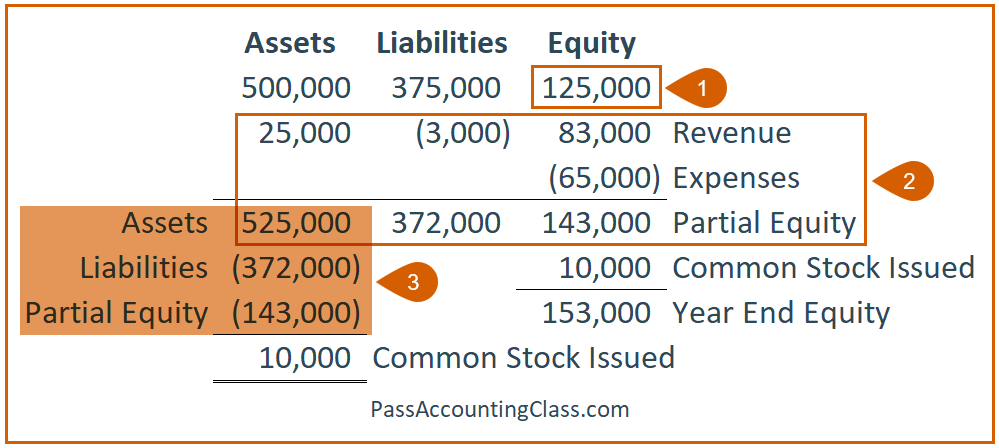For this problem, you need to first solve for the Equity (step 1) by subtracting Liabilities from Assets (500,000 - 375,000). Next, add the information during the year and get ending totals for Assets, Liabilities, and Equity (step 2). Assets = Liabilities + Equity, but at this point our Assets don't equal Liabilities plus Equity. We only have a Partial Equity number. Next we need to subtract Liabilities and Partial Equity from the Assets (step 3). This leaves us with a \$10,000 difference. This difference represents the Common Stock Issued. To prove this, we can add the Common Stock Issued (10,000) to the Partial Equity (143,000) to complete the Accounting Equation. Assets (525,000) = Liabilities (372,000) + Equity (153,000). So the answer to the third question is C.

#### Question 4

Smith Co. issued \$1,000,000 of 5% bonds on July 1, 2020. What was the market rate on the issuance date if the bonds were issued at a discount?
A) 2%
B) 3%
C) 4%
D) 6%

This is another common "theory" accounting question and a lot of times this falls under the category of basic accounting questions. Sometimes you need to memorize accounting information, and this is an example of where you'd want to memorize accounting information. Here's the information you need to learn to solve a problem like this: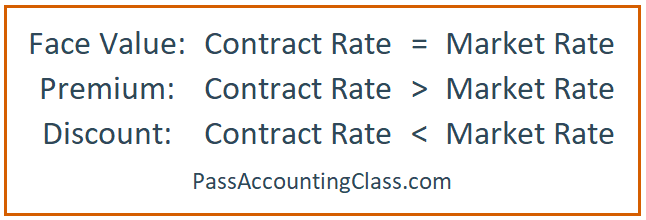Alternatively, sometimes accounting answers can be found by looking at your options. For example, if you look at the answers and look at the information in the problem, you'll notice that 6% is the only percentage that is greater than the 5% mentioned in the question. So, answers A, B, & C are less than the 5%. So the answer to question four is D.

## Question 5

The beginning balance of Allowance for Doubtful Accounts is \$6,000 and the ending balance is \$3,000. During the year, recovered accounts totaled \$2,000 and the estimated bad debt was \$2,500. What amount was written off during the year?
A) \$1,500
B) \$2,500
C) \$3,500
D) \$7,500

Problems like this are best solved using a T-Account. Since this is a Contra-Asset account we need to assume the beginning balance is a normal balance (since we're not told differently). So, we'll put the beginning balance in as a credit (step 1). Next, we'll put the ending balance in (step 2). Next, we'll add the recovered amount (step 3). Then we'll add the estimated bad debt amount (step 4). Finally, we'll add the credits and subtract the ending balance to arrive at the write offs. So, the answer to question five is D.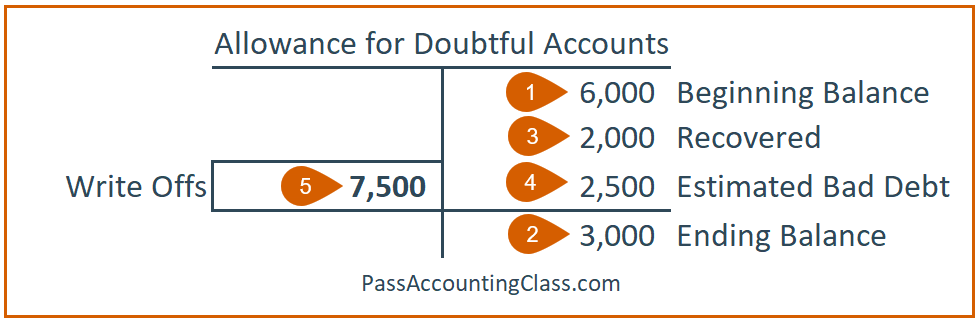How did I know to Credit recovered accounts and estimated bad debt? And how did I know to Debit write offs? Here's where Journal Entries help. If you know the Journal Entries, you'll be able to determine proper Debits and Credits: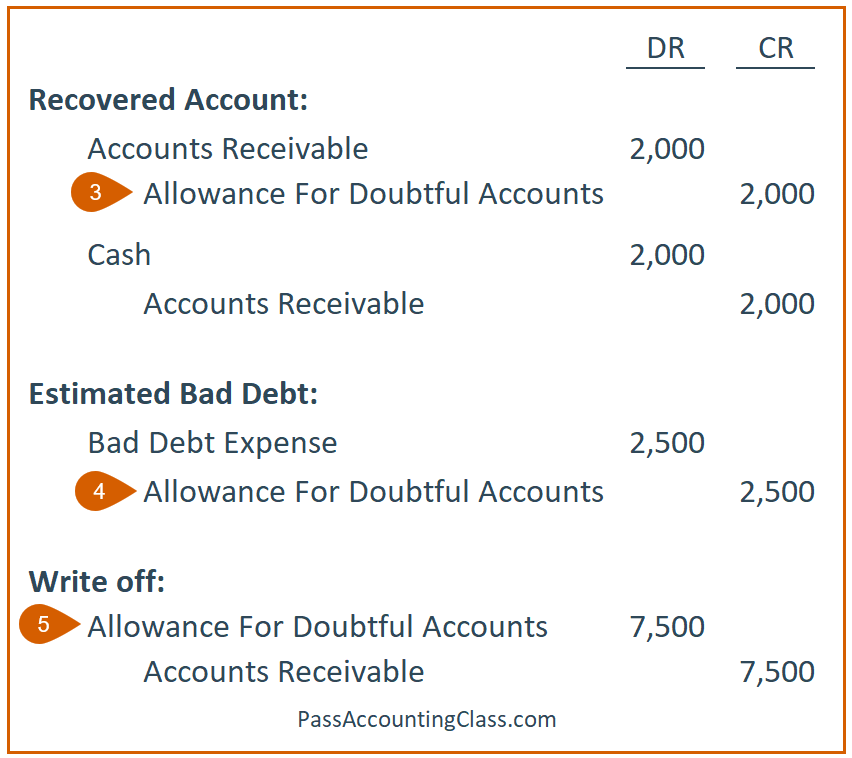There's a lot of information to learn for accounting lass and my goal is to help you pass your accounting class. If you need accounting help, reach out to me! I provide accounting help online to students and can get you back on track quickly. I'm available for one-on-one tutoring here , have a bunch of video training here (scroll to the bottom to see all the lesson topics), or check out some of my free videos on YouTube here.

##### Want to watch how I solve these accounting questions?

Enter your name, email, click the button, and watch the video! You'll see exactly (step-by-step) how I solve these tricky accounting questions.

We won't send you spam. Unsubscribe at any time.